Home Reinforced Concrete Design Manual Design of Beam and Raft Foundation to Eurocode 2 (free PDF...

# Manual Design of Beam and Raft Foundation to Eurocode 2 (free PDF download)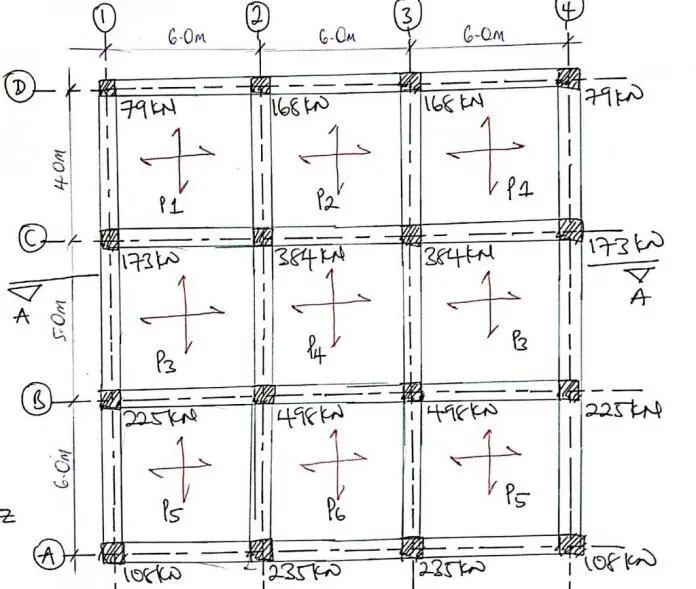In our last post, we considered the analysis of beam and raft foundation of a G+1 building using Staad Pro software. In this particular post we are going to consider the analysis and design of the same foundation using manual calculations according to the principles of the Eurocodes. If you missed the last post, you can read it by clicking on the link below;

An Investigation on the Analysis of Beam and Raft Foundation Using Staad Pro

Also download structural analysis and design of swimming pools and underground water tanks (with excel spreadsheet and video tutorials) by following the link below;
Analysis and design of swimming pools and underground water tanks

The axial forces in the ground floor columns have been presented in Figure 3, and have been used to demonstrate how you can carry out design of beam and raft foundation manually. It is important to point out that the use of ground beams with relatively large depth is usually based on DPC considerations, since the raft slab functions as the ground floor slab too. The raft slab layout and column loads are shown in figure 2 and 3 respectively.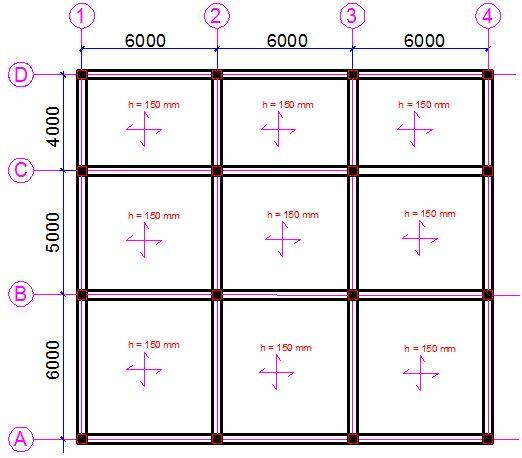Fig 2: Raft foundation layout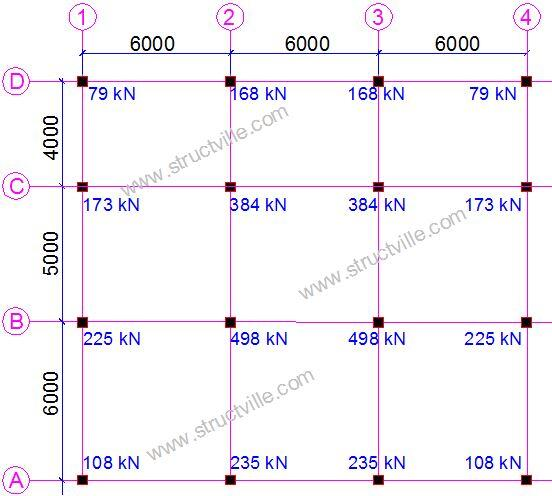Fig 3: Column loads on raft foundation

The schematic diagram of the interaction of a typical beam and raft slab is shown in Figure 4.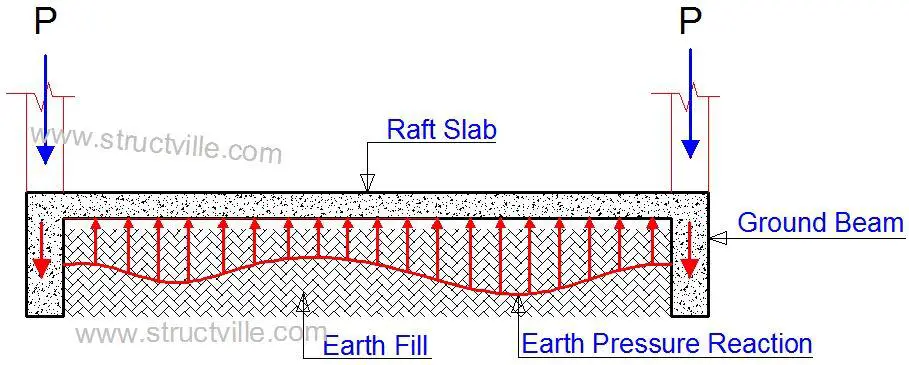Fig 4: Schematic representation of beam and raft slab action

Design Data
Allowable bearing capacity of soil = 40 kPa
Dimension of all columns = 230 x 230 mm
Dimension of all ground beams = 1200 x 250 mm
Thickness of ground floor slab = 150 mm

Analysis of the raft slab by rigid approach
A little consideration of Figure 3 will show that the foundation is loaded is symmetrically in the x-direction, therefore we will compute the eccentricity of the load in the z-direction.

Sum of load on gridline D = 79 + 168 + 168 + 79 = 494 kN
Sum of load on gridline C = 173 + 384 + 384 + 173 = 1114 kN
Sum of load on gridline B = 225 + 498 + 498 + 225 = 1446 kN
Sum of load on gridline A = 108 + 235 + 235 + 108 = 686 kN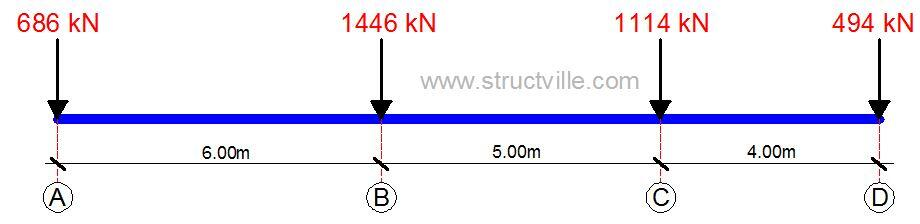Fig 5: Load on the raft slab

Resultant of loads R = 686+ 1446 + 1114 + 494 = 3740 kN

Taking moment about the centroid of the base as shown in Figure 4 above;

M = (-686 x 7.5) – [1446 x (7.5 – 6)] + [1114 x (7.5 – 4)] + (494 x 7.5) = 290 kNm

Eccentricity of the load e = M/R =290/3740 = 0.0775 m to the right hand side

The base dimensions are taken as follows;
L = 6 + 6 + 6 + 0.25 = 18.25 m
B = 6 + 5 + 4 + 0.25 = 15.25m

Pressure distribution Pi = (R/A) ± (6.R.e/LB2)

On substituting and solving;
Pmax = 13.847 kPa
Pmin = 13.028 kPa

These values are way below the allowable bearing capacity of the soil, therefore there is no need to increase the dimensions of the base. Also, due to the close values of Pmax and Pmin, instead of using trapezoidal pressure distribution, we can assume rectangular pressure distribution using the maximum pressure.

Note
Pressure distribution calculation is carried out using SLS load, but we used ULS here. You can convert from ULS to SLS for simple buildings when using Eurocode by using a factor of 1.37 (Ubani, 2017).

Therefore, using a pressure of 13.847 kPa, we can design the raft slabs and the ground beams using the conventional methods which I believe that we are familiar with.

Some screenshots from the manual design is shown below;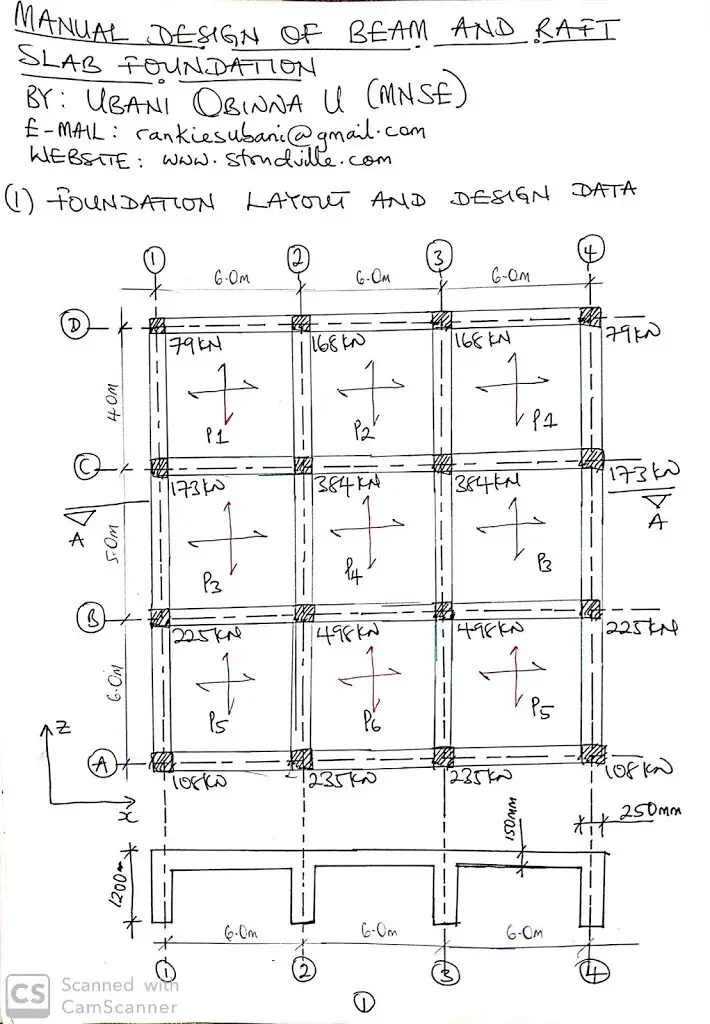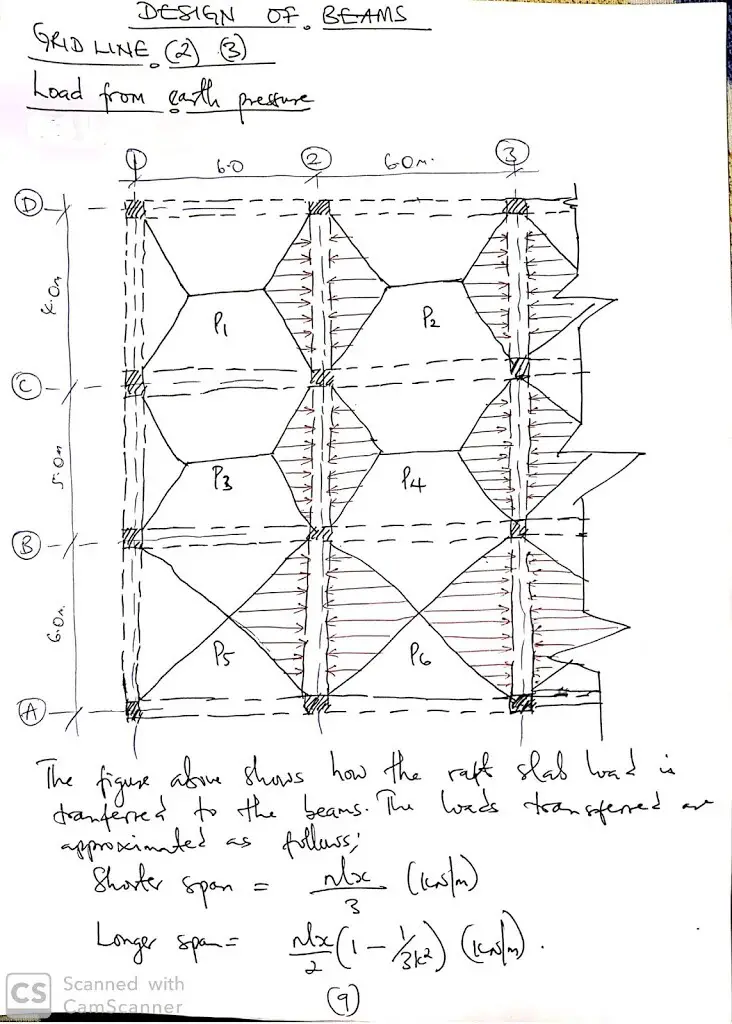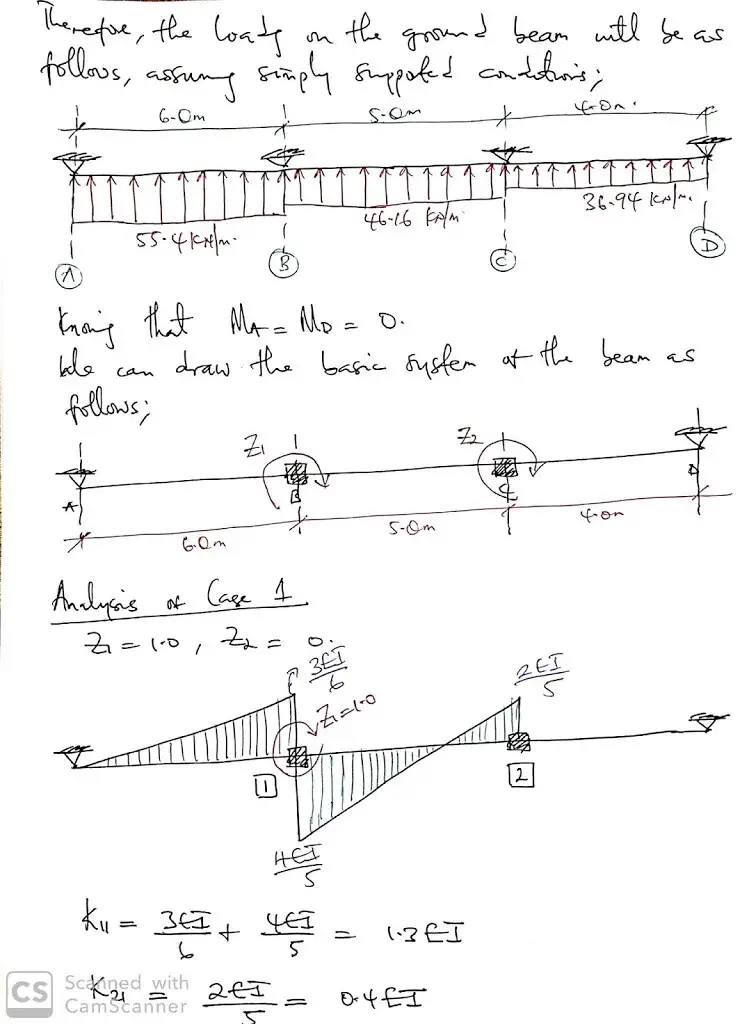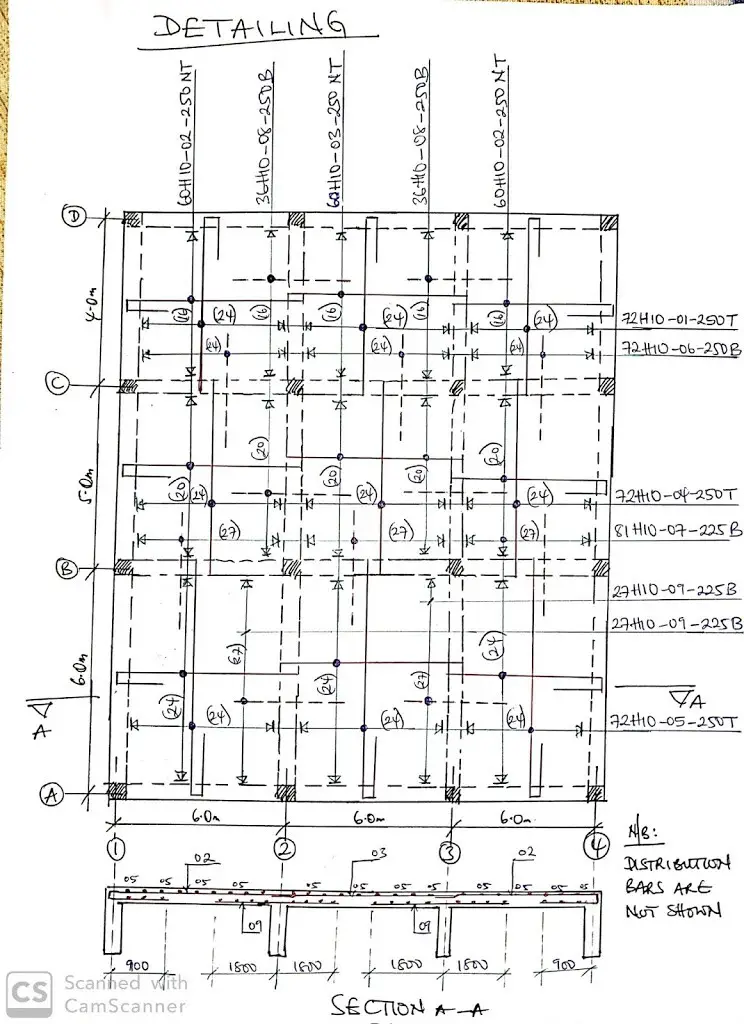Manual Design of Beam and Raft Foundation to Eurocode 2 [PDF]

References
Ubani O.U. (2017): Practical Structural Analysis and Design of Residential Buildings Using Staad Pro V8i, CSC Orion, and  Manual Calculations. Ist Edition, Volume 1. Structville Integrated Services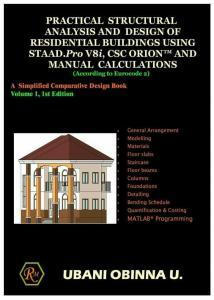1. Hello, I have browsed most of your posts. This post is probably where I got the most useful information for my research. Thanks for posting, maybe we can see more on this. Are you aware of any other websites on this subject.

2. Thanks for this piece

3. Is there an assumption that columns connected to raft foundations don't transmit moments or they are modelled as pinned to the base

•ranks

Definitely they will transmit moment relative to the stiffness of the connection. But the moment is essentially the column moment. So when designing the ground beams, the reinforcement provided for the beam bending moment caters for the moment transferred from beam-column interaction and vice versa.

4.relu59

Thanks !

error: